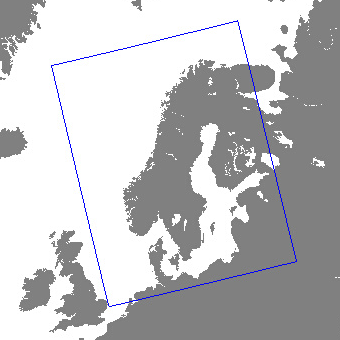# Subseasonal data model

## Data sources

The subseasonal service provides forecasts for the next 21 days. The forecasts cover Norway, Sweden, Finland and Denmark. The extent of the domain is shown in the illustration below.The subseasonal forecast is based on 3 weather prediction models:

1. The MetCoOp Ensemble Prediction System MEPS. MetCoOp is the Meteorological Cooperation on Operational Numeric Weather Prediction (NWP) between Finnish Meteorological Institute (FMI), MET Norway, Swedish Meteorological and Hydrological Institute (SMHI) and Estonian Weather Service. It provides short term forecasts (0 - 60 hours) and has a horizontal resolution of 2.5 kilometers. Forecasts are updated every hour.
2. The atmospheric model Ensemble 15-day forecast from [ECMWF](https://www.ecmwf.int/en/forecasts/datasets/set-iii. Forecasts are updated twice pr day. Horizontal resolution is approximately 10 km. These forecasts are used for days 3 - 15.
3. The Atmospheric Model Ensemble extended forecast from ECMWF. Forecasts are updated once every day. Horizontal resolution is approximately 36 km. These forecasts are used for days 15 - 21.

All forecasts are downscaled to a 1x1 km grid and post-processed to better represent local geographical features. They may therefore differ substantially from the original weather prediction models.

## Variables

{:.note} The list of variables are subject to change and will not be finalized until the product is out of beta.

The variables are aggregations, minima/maxima or average over a time period, either for the next 24 hours or 7 days.

Next 24 hours

Name Unit Description
air_temperature_max celsius maximum air temperature
air_temperature_max_percentile_10 celsius 10th percentile of maximum air temperature (i.e 10% chance maximum will be below this value)
air_temperature_max_percentile_90 celsius 90th percentile of maximum air temperature (i.e 10% chance maximum will be above this value)
air_temperature_min celsius minimum air temperature
air_temperature_min_percentile_10 celsius 10th percentile of minimum air temperature (i.e 10% chance minimum will be below this value)
air_temperature_min_percentile_90 celsius 90th percentile of minimum air temperature (i.e 10% chance minimum will be above this value)
probability_of_frost % probability of air temperature below 0 degrees
probability_of_precipitation % probability of precipitation

Next 7 days

Name Unit Description
air_temperature_daily_high celsius average of daily maximum temperature
air_temperature_daily_low celsius average of daily minimum temperature
days_of_precipitation day number of days with precipitation
precipitation_amount mm precipitation amount
precipitation_amount_percentile_10 mm 10th percentile of precipitation amount (i.e 10% chance it will be below this value)
precipitation_amount_percentile_90 mm 90th percentile of precipitation amount (i.e 10% chance it will be above this value)
probability_of_frost % probability of air temperature below 0 degrees
snowfall_amount cm snowfall amount
snowfall_amount_percentile_10 cm 10th percentile of precipitation amount (i.e 10% chance it will be below this value)
snowfall_amount_percentile_90 cm 90th percentile of precipitation amount (i.e 10% chance it will be above this value)

The variable names are based on the international CF Standard Name vocabulary, which is mandatory for all governmental scientific institutions under the EU INSPIRE directive.